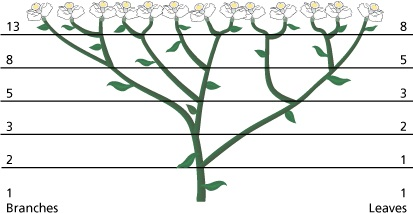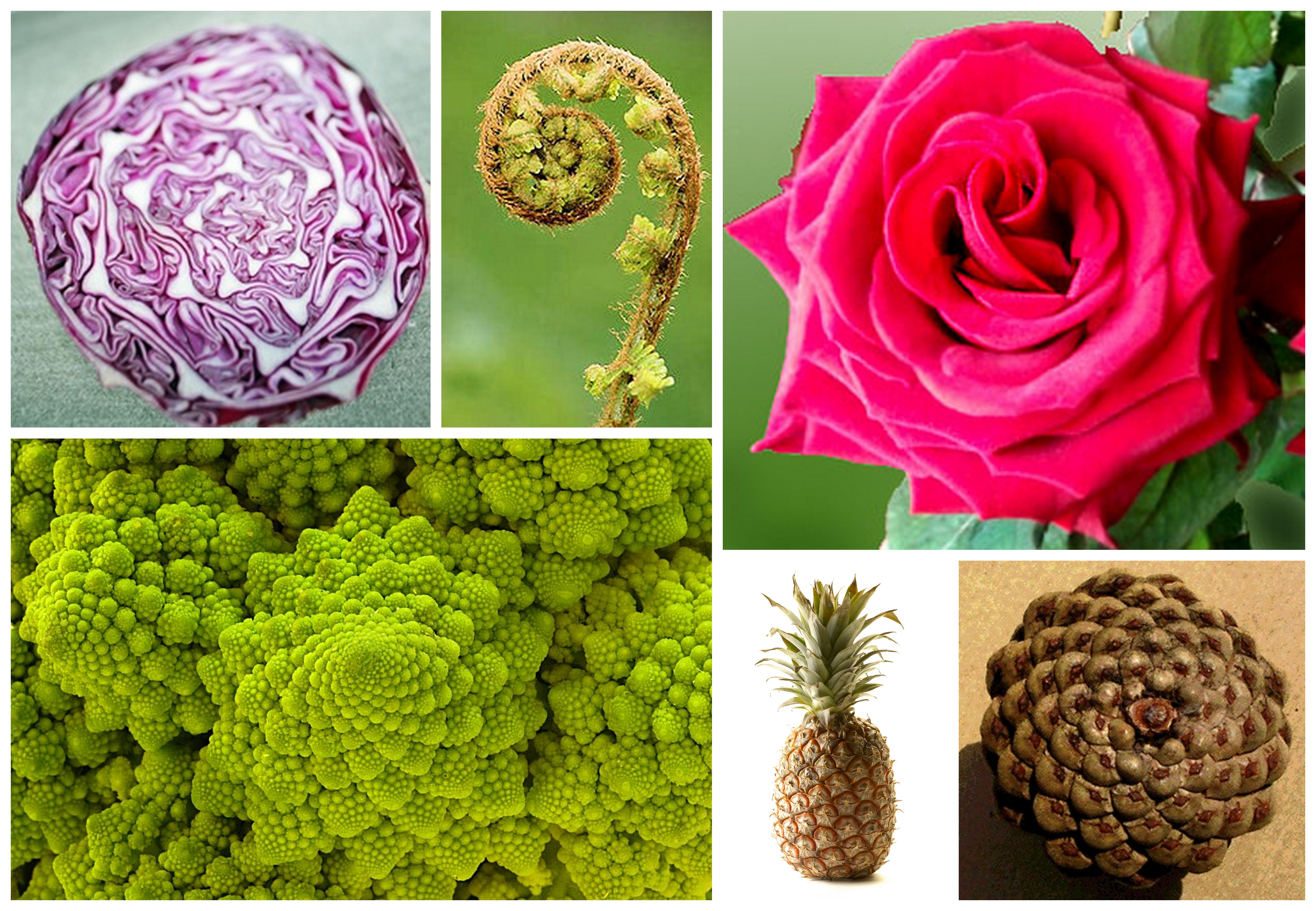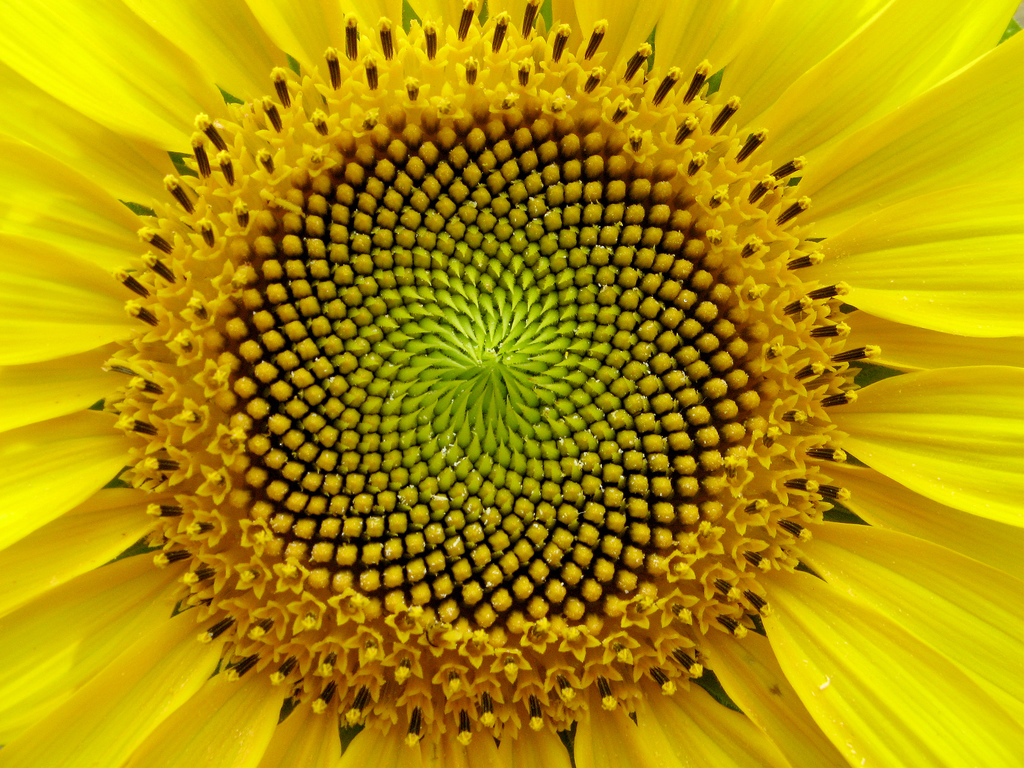#The Language of Nature

They are found everywhere in Nature.  From the leaf arrangement in plants, to the pattern of the petals of a flower, the bracts of a pine cone, or the scales of a pineapple.  The Fibonacci numbers are applicable to the growth of every living thing: a single cell, a grain of wheat, a hive of bees, all of mankind.  From sunflowers to sea shells, the same recurrent mathematical pattern can be observed in Nature, again, and again, and again…

Fibonacci “Leonardo of Pisa” was an Italian mathematician who lived in the Middle Ages.  According to Tobias Dantzig, his father was “a lowly shipping clerk nicknamed ‘Bonaccio’, which, in the idiom of the time, meant a ‘simpleton’.”  Which made ‘Fibonacci,’ the ‘son of a simpleton’…  This couldn’t have been further from the truth.  He was a mathematical genius!

# Fibonacci’s Rabbits

The original problem investigated by Fibonacci in 1202 was about how fast rabbits could breed given ideal circumstances.

Suppose a newly-born pair of rabbits, one male and one female, are placed in a field.  Rabbits can mate at the age of one month, so that at the end of its second month a female can produce another pair of rabbits.  Now, suppose that our rabbits never die and that the female always produces one new pair (one male and one female) every month from the second month on.  The puzzle posed by Fibonacci was the following…

## Fibonacci’s Rabbits

### How many pairs of rabbits will you obtain after one year?

• At the end of the 1st month, they mate, but there is still one only 1 pair.
• At the end of the 2nd month the female produces a new pair, so now there are now 2 pairs of rabbits in the field.
• At the end of the 3rd month, the original female produces a second pair, making 3 pairs in all in the field.
• At the end of the 4th month, the original female has produced yet another new pair, while the female born two months ago produces her first pair also, making 5 pairs.

At the end of the nth month, we see that the number of pairs of rabbits is then equal to the number of new pairs (which is the number of pairs in month n−2), plus the number of pairs alive last month (n−1).

This gives the nth Fibonacci number.

# Honeybees and Sneezewort

## The Bee Ancestry Code

Fibonacci numbers also appear in the description of the reproduction of a population of an idealised honeybees.  The following assumptions are being made that if an egg is laid by an unmated female, it hatches a male or drone bee.  However, if an egg was fertilised by a male, it hatches a female.  Thus, a male bee always has one parent, and a female bee has two.

Therefore, any male bee has 1 parent (1 bee), 2 grandparents, 3 great-grandparents, 5 great-great-grandparents, and so on.  This sequence of numbers of parents is the Fibonacci sequence.  The number of ancestors at each level, Fn, is the number of female ancestors, which is Fn-1, plus the number of male ancestors, which is Fn-2.

This is under the unrealistic assumption that the ancestors at each level are otherwise unrelated.

##The Growth of Sneezewort

Achillea ptarmica or “sneezewort” is a plant that displays the Fibonacci numbers in the number of growing points that it has.

Suppose that when a plant puts out a new shoot, that shoot has to grow two months before it is strong enough to support branching.  If it branches every month after that at the growing point, we get the picture shown here.

What do you see?

Again, whether we consider the branching or the growth of new leaves on sneezewort, the sequence gives the nth Fibonacci number.

# The Fibonacci Sequence

The Fibonacci sequence are the numbers in the following infinite integer sequence:

0, 1, 1, 2, 3, 5, 8, 13, 21, 34, 55, 89, 144, … and so on.

In Mathematical terms, the Fibonacci sequence Fn of numbers is thus defined by a recurrence relation of the form$F_n = F_{n-1} + F_{n-2}$,

where$F_n$ is term number n$F_{n-1}$ is the previous term$(n-1)$,$F_{n-2}$ is the term before that$(n-2)$.

With starting values$F_1 = 1$,$F_2 = 1$  or$F_0 = 0$,$F_1 = 1$.

Or in plain English…

The first two numbers in the Fibonacci sequence are taken to be 1 and 1, or 0 and 1, depending on the chosen starting point of the sequence, and each subsequent number is the sum of the previous two.

## Fibonacci Numbers

For n = 0, 1, 2, …, 20, the first 21 Fibonacci numbers Fn are

n01234567891011121314151617181920
Fn011235813213455891442333776109871597258441816765

# The Golden Ratio

And as luck would have it…

## Golden Number

If you divide each number of the Fibonacci sequence by its predecessor$\frac {F_n}{F_{n-1}}$, you obtain another sequence of numbers that tends to a number$\phi$.$\phi = \frac {1+\sqrt{5}}{2} \approx 1.62$

That number$\phi$ φ is known as the golden number.

The golden ratio or golden mean (only one of the ways the number has been called through the ages) has a special significance in Mathematics and the Arts.

Ever since Euclid (fl. 300 BC), mathematicians have studied the golden ratio for its frequent appearance in geometry.  Scholars speculated that Leonardo da Vinci (1452-1519) incorporated the golden ratio in his paintings.

German astronomer Johannes Kepler (1571-1630) proved that the golden ratio is the limit of the ratio of consecutive Fibonacci numbers, and described the golden ratio as a “precious jewel”:

“Geometry has two great treasures: one is the Theorem of Pythagoras, and the other the division of a line into extreme and mean ratio; the first we may compare to a measure of gold, the second we may name a precious jewel.”

French composer Erik Satie (1866–1925) used the golden ratio in several of his pieces.  So did Debussy (1862–1918).

The properties of the golden ratio include its appearance in the dimensions of a regular pentagon and in a golden rectangle, which can be cut into a square and a smaller rectangle with the same aspect ratio.

# The Golden Spiral Approximationgolden spiral can be approximated by drawing circular arcs connecting the opposite corners of squares in the Fibonacci tiling.  This spiral uses squares of sizes 1, 1, 2, 3, 5, 8, 13, 21, and 34.

We start with two small squares of size 1 next to each other.  On top of both of these, we then draw a square of size 2 (=1+1).  Now we draw a new square – touching both a unit square and the latest square of side 2 – thereby having sides 3 units long, and then another touching both the 2-square and the 3-square (which has sides of 5 units).

We can continue this by adding squares around the picture, each new square having a side which is as long as the sum of the latest two square’s sides.

This set of squares (or rectangles) whose sides are two successive Fibonacci numbers in length and which are composed of squares with sides which are Fibonacci numbers, are called the Fibonacci rectangles.

The spiral-in-the-squares makes a line from the centre of the spiral increase by a factor of the golden number in each square.

So, the points on the golden spiral are

1.618 times as far from the centre after one quarter of a turn.

In a whole turn, the points on a radius out from the centre are 1.6184 = 6.854 times further out than when the curve last crossed the same radial line.

The spiral is not a true mathematical spiral (since it is made up of fragments which are parts of circles and does not go on getting smaller and smaller) but it is a good approximation to a kind of spiral that does appear often in Nature.

#Sea Shells and Flower Seeds

Such spirals are found in the shape of the shells of snails and those of sea shells, as well as in the arrangement of seeds on flowering plants too.

Pine cones and fir cones also show the numbers.  Some coniferous trees show these numbers in the bumps on their trunks.  And palm trees have the numbers in the rings on their trunks.

Many plants display the Fibonacci numbers in the arrangement of their leaves and petals around the stem, including succulents, daisies and sunflowers.

# Phyllotaxis

Why do these arrangements occur?  Surely, plants do not know about this sequence.  No, I grant you they don’t.  For that matter, neither do rabbits.  Plants follow these recurrent patterns because that is how they grow in the most efficient ways.

When it comes to leaf arrangement (phyllotaxis), it may be related to optimising the space required for each leaf to grow, or the average amount of light falling on each one.  Even a tiny advantage would come to dominate, over many generations.  In the case of close-packed leaves in cabbages and succulents, the correct arrangement may be crucial for space availability.

## Linking Mathematical Patterns and Nature – Turing’s Sunflowers Project

The largest ever research project into mathematical patterns in flowers proved a link between number sequences and nature, Manchester scientists said.  Hundreds of volunteers worldwide got involved, growing sunflowers as part of a project led by Manchester’s Museum of Science and Industry and Manchester University.

The research proved conclusively that most spirals of seeds in the sunflowers conformed to patterns.

Data from 557 sunflowers from seven countries was collected for the Turing’s Sunflowers Project, set up to celebrate the centenary of the mathematician’s birth, and growers kept video diaries about their flowers’ progress.   Scientists aimed to test the theory of Manchester-based computer pioneer Alan Turing, who died in 1954.

It showed 82% of the flowers conformed to complex structures including the mathematical Fibonacci sequence – where each number is the sum of the previous two.Scientists said this proves that Mathematics is an integral part of Nature.  It could provide clues to help biologists understand how plants develop.

Professor Jonathan Swinton, a computational biologist, said: “It’s the most comprehensive information we have so far on Fibonacci numbers in sunflowers and we have proved what Alan Turing observed when he looked at a few sunflowers in his own garden in Wilmslow.  Now, we need to work together with biologists to understand the wider implications of different number patterns for plant growth.”

The appearance of patterns in the phyllotaxis – the arrangement of leaves, stems or seeds – has been studied by many well-known scientists, including Leonardo Da Vinci.  Turing, who directed the computing laboratory at the University of Manchester, died before he could test his theory about the Fibonacci sequence.

The results of the project, announced at the 2012 Manchester Science Festival, were published in a scientific paper so further studies can explore the reasons why number patterns occur in Nature.